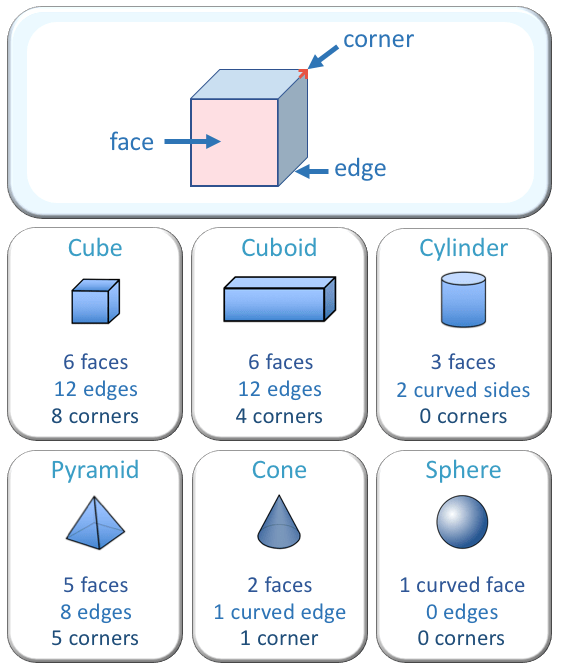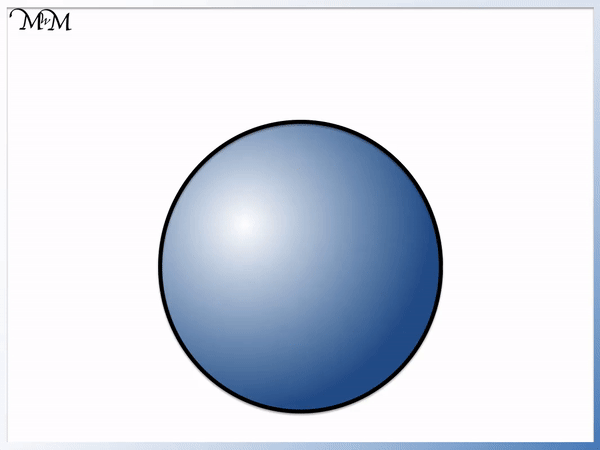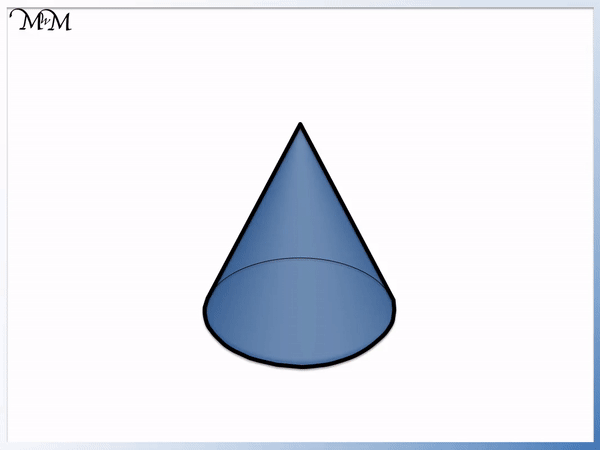# Names of 3D Shapes

Names of 3D Shapes• 3D means three-dimensional
• 3D shapes can be held and picked up
• 3D shapes have faces, edges and corners (or vertices)
• Faces are the outside surfaces of a 3D shape
• Edges are the straight lines where two faces meet
• Corners (or vertices) are where one or more edges meet• This is a cube.
• A cube has 6 square faces.
• Therefore each of the 6 faces are the same
• A cube has 12 edges that are all the same length.
• A cube has 8 corners.
• Some common everyday objects that are cubes are: a dice, a Rubik’s cube and an ice cube.# What are the Names of 3D Shapes?

In this lesson we are learning the names of 3D shapes and looking at examples of some common everyday objects that have these shapes.

3D means three-dimensional. A three-dimensional shape has depth as well as length and width.

If you can hold or pick up an object, then it is 3D. This means that all real objects are actually made from 3D shapes.

We will look at the following common 3D shapes:

• Cubes
• Cuboids
• Spheres
• Cylinders
• Pyramids
• Cones

The first shape we will look at is a cube.All of a cube’s surfaces are the same and all of a cube’s edges are the same length.

A cube will not roll, it will slide.

When teaching shapes it is useful to have some real life examples to show.

Dice are cubes, a Rubik’s cube is a cube and an ice cube is a cube.

Can you think of any more?

Our next shape is a Cuboid.

It is very similar to a Cube, but the difference is that it is longer than it is wide, whereas every length of a cube is the same.A cuboid is shaped like a box and some people describe it as a 3D rectangle.

Some examples of cuboids are bricks, either house bricks or toy bricks.

A cuboid will also slide instead of rolling as it does not have any curved surfaces.

Below is a sphere.

A sphere is a ball shape, however we do not call it a ‘ball’.

It might be perceived as a 3D circle, however we do not call it a circle’ either.A sphere has one completely round face and will roll when pushed in any direction.

All types of ball are spheres, such as footballs and tennis balls.

The next 3D shape is called a cylinder.

A cylinder has a circle on its top surface and a circle on its base, along with one continuous curved side.A cylinder is similar to a sphere in that it rolls, however it is different because it has two edges.

When teaching the names of 3D shapes it is important to be clear on the difference between similar shapes such as a cylinder and a sphere.

It can help to take real life examples and in this example we can feel the edges on the cylinder and compare this to the lack of edges on a sphere.

Common everyday objects that are cylinders are tins and batteries.

The next 3D shape we will look at is a pyramid.It is similar in appearance to a cone below.A pyramid is different to a cone in a couple of ways.

A cone has a circular base, whereas a pyramid does not. In the example we have shown above, the pyramid has a rectangular base, however it can have other shaped bases too such as a triangle or a hexagon.

A cone will roll and a pyramid will not. This is because a cone has a curved face, whereas a pyramid has flat surfaces.Now try our lesson on What is the Volume of a Cuboid? where we learn how to find the volume of a cuboid.